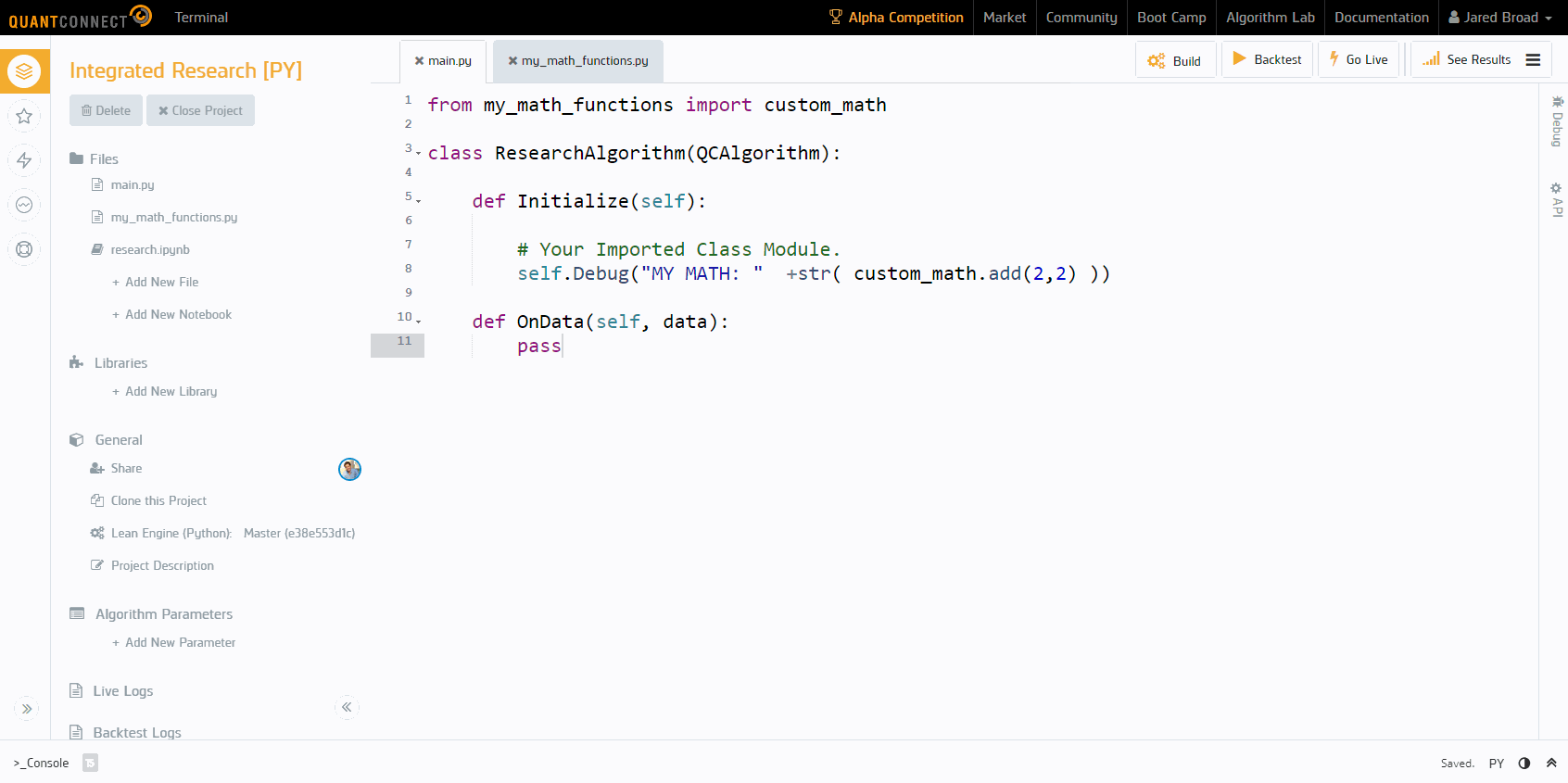## From Research To Production TutorialsResearch to Production is a series of blog post tutorials that make use of QuantConnect’s deep integration with Jupyter Lab to give you a powerful new tool for your alpha research. With this series, you can see how to quickly take your ideas from the research notebook and implement them in an algorithm. You can use QuantConnect’s research environment in Python and C#.

### Read and Clone a Tutorial

The series covers implementations of statistical and machine learning concepts with code snippets and a template algorithm to clone. You can start with any topic:

1. Mean Reversion: use the research notebook to calculate the spread between an asset’s price and its historical mean from the past 30 days. Then, calculate the standard deviation and determine which assets have moved more than one standard deviation below their average price. Take advantage of the mean reversion theory and take long positions.
2. Random Forest Regression: train a supervised learning random forest regression model to identify important features of our datase. Use the model weights to build a tradeable portfolio.
3. Uncorrelated Assets: build a portfolio that can be more diversified and resilient to extreme market events by looking for uncorrelated assets. When combined with other indicators and data sources, the diverse portfolio becomes the foundation for building a robust algorithm.
4. Kalman Filter and Pairs Trading: implement a strategy using a Kalman filter to predict pairs trading hedge ratios. The Kalman filter takes measurements over time and estimates unknown variables using a joint probability distribution of the variables for each timeframe. It can be used instead of a moving average indicator and could be a great next step in your algorithm design.
5. Stationary Processes and Z-Scores: implement two concepts that improve an Alpha’s ability to forecast. Test for stationarity with the Augmented Dickey-Fuller (ADF) test and normalize the data based on z-scores.
6. Principal Component Analysis: implement a strategy using Principal Component Analysis to identify the dimensions of data, or number of components, that contribute most to data variance. We can use PCA-transformed data to build regression models, reduce the number of factors in our model to avoid overfitting, and make predictions.
7. Hidden Markov Models: use Hidden Markov Models to represent the market shift from bull to bear and to predict the market state. A Markov process is stochastic where the possibility of switching to another state depends only on the current state of the model.
8. Long Short-Term Memory: apply a neural networks with a “Long-Short-Term Memory” to a time-series prediction. By having a long memory, LSTM models are better able to capture market outliers such as recessions with less overfitting.

You can learn QuantConnect’s API through their coding labs and with this free Udemy course.### By: Sherry Yang

02.03.2020Back to Blog

## Related Articles

algorithmic trading By: Jared Broad • 07.06.2021 bond yields By: Derek Melchin • 01.06.2021 financial times By: Derek Melchin • 01.06.2021 bitcoin By: Derek Melchin • 28.05.2021 christmas By: Derek Melchin • 28.05.2021